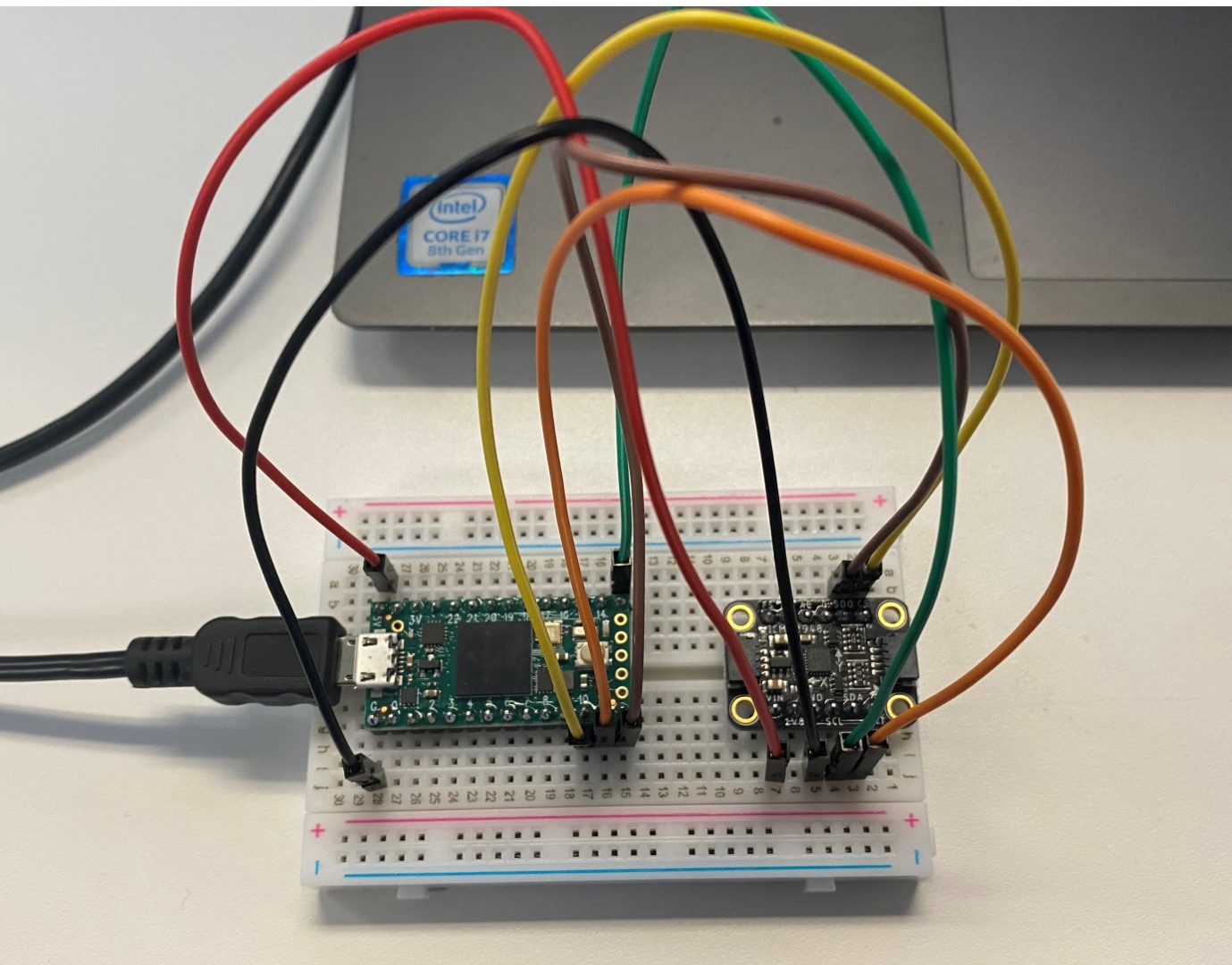## Project Idea

I generate a good number of 3D plots for work and other projects, and for a while I’ve wanted to make a physical device that acts as a “virtual camera” that would let me fly around the 3D data virtually. In a fully featured end state, this would basically be a simple virtual reality setup – there would be a volume of space at my desk that represents the volume of the 3D plot, and I could move the device around in it to zoom in and look at data from any angle.

## Hacking matplotlib

Unfortunately, there was immediately a roadblock. My favorite (and probably yours too!) Python plotting library matplotlib only gives you two angles to define your viewpoint in 3D plots: elevation and azimuth. This lets you move the camera location to any position around the plot, but the Z axis is always going to be “up”. In 3D space we need 3 angles to define an arbitrary orientation, and here we are missing a “roll” angle that rotates the camera about the direction it’s pointing. There were basically two options to get around this. One, ditch matplotlib and use another visualization library like PyVista or plotly that handles 3D plots better. Or two, add the functionality I needed to matplotlib. This decision was easy – why learn another library when you could spend ten times as long hacking the one you know? And never let an opportunity to give back to open source go to waste!

Once I got the roll angle working, I submitted it as a pull request to the matplotlib official repository. This was my first time contributing to a major open source project, and while it was a decent bit of effort to get code quality and test coverage up to the standards of a project that sees 26 million downloads every month, I found the chance to share useful features with that many users incredibly rewarding. Matplotlib is the de-facto default of plotting in python, and python is the de-facto default programming language of education, science, and frankly programming overall. And beyond giving it a third view angle, I found that once I had a development version set up the ball had started rolling and I kept finding things to fix and add in.

So I’m happy to share these new features in matplotlib 3.6, which is out now!

Plus these, hopefully coming in matplotlib 3.7:

## Hardware Setup

But back to the original project. To get the orientation for my virtual camera, I needed an inertial measurement unit (IMU). The IMU contains an accelerometer, magnetometer, and gyroscope, which fuse their data to determine the orientation of the device in 3D space. The accelerometer could be used to calculate the change in position as I move it around, but unfortunately all accelerometers will have a small bias in one direction, and this will cause the estimated position to drift over time. A source of absolute position is needed to counteract this drift, which is why an IMU is often paired with GPS, cameras, radar, lidar, or sonar in real-world vehicles and robots. Right now I think that this would add a little too much scope to the project, so I shelved the idea of flying the virtual camera around in 3D space and decided to just have the IMU control the view angle of the plot.

The IMU I chose was a ICM-20948, which is the current upgrade to the popular MPU-9250, and it does all the sensor fusion onboard so I don’t have to worry about implementing a Kalman filter. This gets wired up on a breadboard to a Teensy 4.0 microcontroller (essentially a more powerful Arduino), which reads the output of the IMU and sends the orientation quaternions to my laptop.The Teensy 4.0 (left) wired to the ICM-20948 IMU (right) via an SPI connection, all connected to my laptop over USB

## Quaternion Math

Quaternions are the right way to represent orientations and rotations in 3D space, and check out this interactive video series by 3blue1brown for a great introduction. However, quaternions are not supported by default in numpy (see the numpy-quaternion package for adding this functionality) and so in the interest of maintaining backwards compatibility and not adding dependencies, I did not update matplotlib to use them internally. However, we can convert the quaternions from the IMU to matplotlib’s Euler angles in a straightforward manner.

The equations to do this can be found in the paper Diebel, James “Representing Attitude: Euler Angles, Unit Quaternions, and Rotation Vectors” (2006) as Sequence (3, 2, 1), with azimuth as φ, elevation as -θ, and roll as ψ. To convert from the camera frame to the axes frame, the rotation matrices are applied in the (right-multiplied) order R = Rz(φ)Ry(θ)Rx(ψ), and to convert from the axes to the camera frame this rotation matrix is inverted.

For anyone who wants to do a similar project, the core of the calculation is below. Feel free to reuse!

``````import numpy as np
import matplotlib.pyplot as plt  # matplotlib >= 3.6.0
import quaternion  # package numpy-quaternion

# See Diebel, James "Representing Attitude: Euler Angles, Unit Quaternions, and Rotation Vectors" (2006)
# Sequence (3, 2, 1)
def quat_to_elev_azim_roll(q, angle_offsets=(0, 0, 0)):
q0, q1, q2, q3 = q.w, q.x, q.y, q.z
phi = np.arctan2(-2*q1*q2 + 2*q0*q3, q1**2 + q0**2 - q3**2 - q2**2)
theta = np.arcsin(2*q1*q3 + 2*q0*q2)
psi = np.arctan2(-2*q2*q3 + 2*q0*q1, q3**2 - q2**2 - q1**2 + q0**2)
return elev, azim, roll

def elev_azim_roll_to_quat(elev, azim, roll, angle_offsets=(0, 0, 0)):
q0 = np.cos(phi/2)*np.cos(theta/2)*np.cos(psi/2) - np.sin(phi/2)*np.sin(theta/2)*np.sin(psi/2)
q1 = np.cos(phi/2)*np.cos(theta/2)*np.sin(psi/2) + np.sin(phi/2)*np.sin(theta/2)*np.cos(psi/2)
q2 = np.cos(phi/2)*np.sin(theta/2)*np.cos(psi/2) - np.sin(phi/2)*np.cos(theta/2)*np.sin(psi/2)
q3 = np.cos(phi/2)*np.sin(theta/2)*np.sin(psi/2) + np.sin(phi/2)*np.cos(theta/2)*np.cos(psi/2)
q = np.quaternion(q0, q1, q2, q3)
return q

q = np.quaternion(1, 0, 0, 0)
angles_init = (0, 0, 0)
elev, azim, roll = quat_to_elev_azim_roll(q, angles_init)